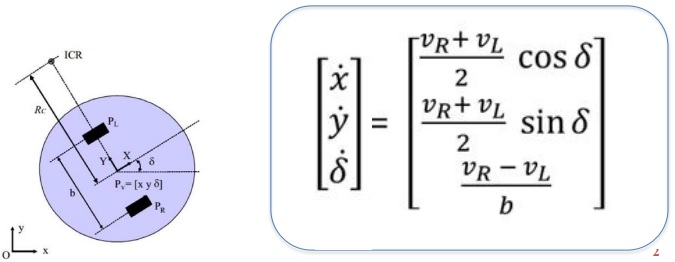# Mobile Robot Control based on Differential Drive (DD)

## Description

The Control Problems
➢ Speed Control
• Given desired speed of the robot, vxd, vyd, calculate control inputs, i.e., speeds of driving wheels.

• For example, for the DD robot below, if we want the reference point of the robot to move at speed (vxd, vyd), what is the corresponding vR, vL?➢ Inputs: vR, vL (Speed of wheels)
➢ Outputs: x, y, delta
➢ Recall the fact that the DD robot cannot take side slip and thus the corresponding (vxd, vyd) along the wheel axis direction can not be achieved.

Overall, the kinematics of (xr, yr) is:whereWe thus immediately get the control input (vRd, vLd) to achieve (vxd, vyd),➢ Initial positions and heading: x=0;y=0;delta=0
➢ Robot parameters: b=0.1;d=0.05;
➢ vxd=0.3, vyd=-0.1;➢ Initial positions and heading: x=0;y=0;delta=0
➢ Robot parameters: b=0.1;d=0.05;
➢ vxd=0.3, vyd=-0.1;➢ Initial positions and heading: x=0;y=0;delta=0
➢ Robot parameters: b=0.1;d=0.05;
➢ vxd=0.3*sin(5*t), vyd=-0.1;## Reviews

There are no reviews yet.

SKU: CTRDDROBXA34 Category: